# Angles

## Activities

Estimating AnglesEstimate the size of the given acute angles in degrees.

Measuring Angles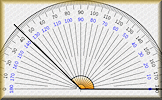Measure the size of the given angles to within two degrees of their actual value.

Angle Points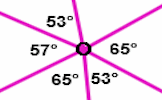Apply the properties of angles at a point, angles on a straight line and vertically opposite angles.

Pie Charts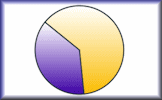Develop the skills to construct and interpret pie charts in this self-marking set of exercises.

Angles in a Triangle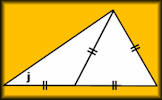A self marking exercise involving calculating the unknown angle in a triangle.

Angle Parallels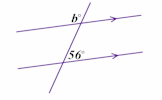Understand and use the relationship between parallel lines and alternate and corresponding angles.

Angle ChaseFind all of the angles on the geometrical diagrams.

Scale Drawings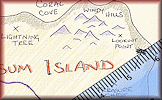Measure line segments and angles in geometric figures, including interpreting scale drawings.

Online LogoAn online version of the Logo programming language with 30 mathematical challenges.

Polygon Angles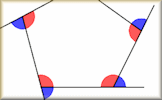A mixture of problems related to calculating the interior and exterior angles of polygons.

Circle Theorems ExerciseShow that you understand and can apply the circle theorems with this self marking exercise.

Circle Theorem PairsA pairs game based around ten theorems about the angles made with chords, radii and tangents of circles.

Snooker AnglesAn online game for one or two players requiring an ability to estimate angles as bearings.

Angles MixedFind the unknown angles by using the basic angle theorems.

Proof of Circle TheoremsArrange the stages of the proofs for the standard circle theorems in the correct order.

Geometry ToolboxCreate your own dynamic geometrical diagrams using this truly amazing tool from GeoGebra.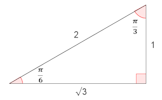A self-marking exercise on finding the exact values of sine, cosine and tangent of special angles given in radians.

## Investigations

Tessellations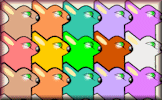Drag the shapes onto the canvas to create tessellating patterns then try the tessellations quiz.

## Videos

This is the main Transum help video on Angles.

Measuring Angles Video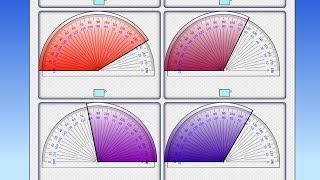Learn how to use a protractor to measure angles of any size. This video is to help you do the online, self-marking exercise.

Angle Points Video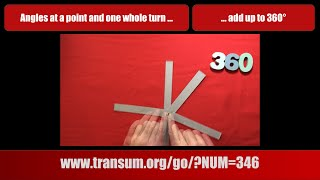See a demonstration of angles in a full turn around a point, angles together on a straight line and vertically opposite angles.

Angles in a Triangle Video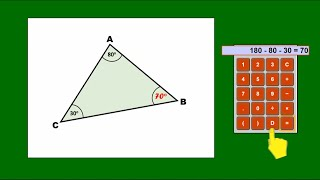A reminder of how to use the fact that the angles in a triangle sum to 180 degrees to find the size of unmarked angles in triangular diagrams.

Angle Properties Song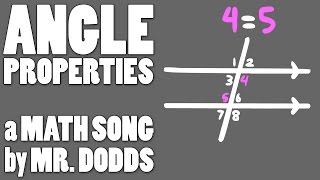Polygon Angles Video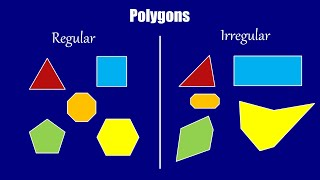A concise reminder about angles, both interior and exterior, in regular and irregular polygons.

Angles in Depth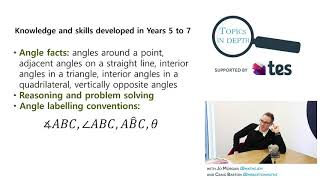Craig Barton and Jo Morgan help you and your class understand angles through the means of kung fu.

The 50 Cent Riddle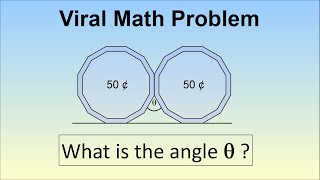A 50 cent coin has 12 equal sides. If you place two coins next to each other on a table, what is the angle formed between the two coins?

Ruler and Compass Construction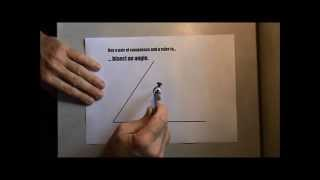A demonstration of standard ruler and compass constructions

## Visual Aids

Kite Maths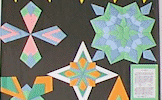Can you make a kite shape from a single A4 size sheet of paper using only three folds?

Angle Theorems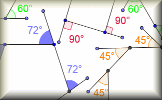Diagrams of the angle theorems with interactive examples.

Polygon Angles Animation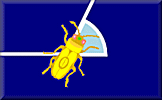Barbara Bug walks around a regular hexagon turning through each of the exterior angles as she goes.

Angle Theorem Kim's Game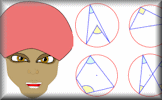A memory game to be projected to help the whole class revise the circle angle theorems.

Plane BearingsA visual aid designed to help pupils estimate three figure bearings.

Circle Theorems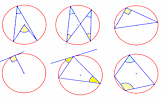Diagrams of the circle theorems to be projected onto a white board as an effective visual aid.

Geometry ToolboxCreate your own dynamic geometrical diagrams using this truly amazing tool from GeoGebra.

## StartersAir Traffic Control
Work out which aircraft are in danger of colliding from their positions and direction of travel. An exercise in understanding bearings.Angle Estimates
Estimate the sizes of each of the angles then add your estimates together.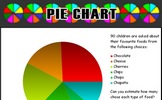Pie Chart
An exercise in estimating what the sectors of a pie chart represent.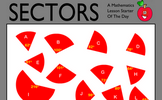Sectors
Work out which sectors fit together to make complete circles. Knowledge of the sum of the angles at a point will help find more than one correct solution to this puzzle.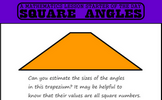Square Angles
Find a trapezium, a triangle and a quadrilateral where all of the angles are square numbers.

31 items are currently in this category.

Teachers might find the complete Angles Topic List useful.

Pupils should understand that angles represent an amount of turning and be able to estimate the size of angle. When constructing models and drawing pupils should be able to measure and draw angles to the nearest degree and use appropriate language associated with angles.

Pupils should know the angle sums of polygons and that of angles at a point and on a straight line. They will learn about angles made in circles by chords, radii and tangents and recognise the relationships between them.

Pupils will work with angles using trigonometry, transformations and bearings. In exams pupils are often instructed that while non-exact answers should be given to three significant figures, angle answers should be given to one decimal place.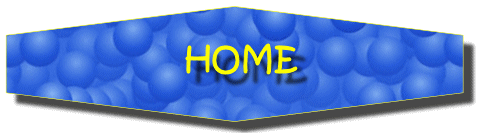For All: